## Free Statistics

of Irreproducible Research!

Author's title
Author*The author of this computation has been verified*
R Software Modulerwasp_edauni.wasp
Title produced by softwareUnivariate Explorative Data Analysis
Date of computationWed, 01 Dec 2010 15:00:34 +0000
Cite this page as followsStatistical Computations at FreeStatistics.org, Office for Research Development and Education, URL https://freestatistics.org/blog/index.php?v=date/2010/Dec/01/t1291215533guzw2bonxoy6pgu.htm/, Retrieved Thu, 06 Oct 2022 00:48:09 +0000
Statistical Computations at FreeStatistics.org, Office for Research Development and Education, URL https://freestatistics.org/blog/index.php?pk=104045, Retrieved Thu, 06 Oct 2022 00:48:09 +0000
QR Codes:Original text written by user:
IsPrivate?No (this computation is public)
User-defined keywords
Estimated Impact137
Family? (F = Feedback message, R = changed R code, M = changed R Module, P = changed Parameters, D = changed Data)
-     [Univariate Explorative Data Analysis] [time effect in su...] [2010-11-17 08:55:33] [b98453cac15ba1066b407e146608df68]
-    D  [Univariate Explorative Data Analysis] [Run sequency plot...] [2010-11-20 14:46:56] [95e8426e0df851c9330605aa1e892ab5]
-           [Univariate Explorative Data Analysis] [] [2010-12-01 15:00:34] [6d73806852b9f5b8ac8b27fc8f7b83c4] [Current]
-             [Univariate Explorative Data Analysis] [] [2010-12-03 17:26:41] [5e7b9ab9ddedd2d2f5ce6c303ba3ebe3]
-   PD        [Univariate Explorative Data Analysis] [run sequence plot...] [2011-11-24 10:08:43] [ca5872d1d4d784184b94263c274137e3]
- RMPD        [Multiple Regression] [tre] [2011-11-24 10:14:29] [ca5872d1d4d784184b94263c274137e3]
Feedback Forum

Post a new message
Dataseries X:
11
7
17
10
12
12
11
11
12
13
14
16
11
10
11
15
9
11
17
17
11
18
14
10
11
15
15
13
16
13
9
18
18
12
17
9
9
12
18
12
18
14
15
16
10
11
14
9
12
17
5
12
12
6
24
12
12
14
7
13
12
13
14
8
11
9
11
13
10
11
12
9
15
18
15
12
13
14
10
13
13
11
13
16
8
16
11
9
16
12
14
8
9
15
11
21
14
18
12
13
15
12
19
15
11
11
10
13
15
12
12
16
9
18
8
13
17
9
15
8
7
12
14
6
8
17
10
11
14
11
13
12
11
9
12
20
12
13
12
12
9
15
24
7
17
11
17
11
12
14
11
16
21
14
20
13
11
15
19

 Summary of computational transaction Raw Input view raw input (R code) Raw Output view raw output of R engine Computing time 26 seconds R Server 'George Udny Yule' @ 72.249.76.132

\begin{tabular}{lllllllll}
\hline
Summary of computational transaction \tabularnewline
Raw Input & view raw input (R code)  \tabularnewline
Raw Output & view raw output of R engine  \tabularnewline
Computing time & 26 seconds \tabularnewline
R Server & 'George Udny Yule' @ 72.249.76.132 \tabularnewline
\hline
\end{tabular}
%Source: https://freestatistics.org/blog/index.php?pk=104045&T=0

[TABLE]
[ROW][C]Summary of computational transaction[/C][/ROW]
[ROW][C]Raw Input[/C][C]view raw input (R code) [/C][/ROW]
[ROW][C]Raw Output[/C][C]view raw output of R engine [/C][/ROW]
[ROW][C]Computing time[/C][C]26 seconds[/C][/ROW]
[ROW][C]R Server[/C][C]'George Udny Yule' @ 72.249.76.132[/C][/ROW]
[/TABLE]
Source: https://freestatistics.org/blog/index.php?pk=104045&T=0

Globally Unique Identifier (entire table): ba.freestatistics.org/blog/index.php?pk=104045&T=0

As an alternative you can also use a QR Code:

The GUIDs for individual cells are displayed in the table below:

 Summary of computational transaction Raw Input view raw input (R code) Raw Output view raw output of R engine Computing time 26 seconds R Server 'George Udny Yule' @ 72.249.76.132

 Descriptive Statistics # observations 159 minimum 5 Q1 11 median 12 mean 12.8553459119497 Q3 15 maximum 24

\begin{tabular}{lllllllll}
\hline
Descriptive Statistics \tabularnewline
# observations & 159 \tabularnewline
minimum & 5 \tabularnewline
Q1 & 11 \tabularnewline
median & 12 \tabularnewline
mean & 12.8553459119497 \tabularnewline
Q3 & 15 \tabularnewline
maximum & 24 \tabularnewline
\hline
\end{tabular}
%Source: https://freestatistics.org/blog/index.php?pk=104045&T=1

[TABLE]
[ROW][C]Descriptive Statistics[/C][/ROW]
[ROW][C]# observations[/C][C]159[/C][/ROW]
[ROW][C]minimum[/C][C]5[/C][/ROW]
[ROW][C]Q1[/C][C]11[/C][/ROW]
[ROW][C]median[/C][C]12[/C][/ROW]
[ROW][C]mean[/C][C]12.8553459119497[/C][/ROW]
[ROW][C]Q3[/C][C]15[/C][/ROW]
[ROW][C]maximum[/C][C]24[/C][/ROW]
[/TABLE]
Source: https://freestatistics.org/blog/index.php?pk=104045&T=1

Globally Unique Identifier (entire table): ba.freestatistics.org/blog/index.php?pk=104045&T=1

As an alternative you can also use a QR Code:

The GUIDs for individual cells are displayed in the table below:

 Descriptive Statistics # observations 159 minimum 5 Q1 11 median 12 mean 12.8553459119497 Q3 15 maximum 24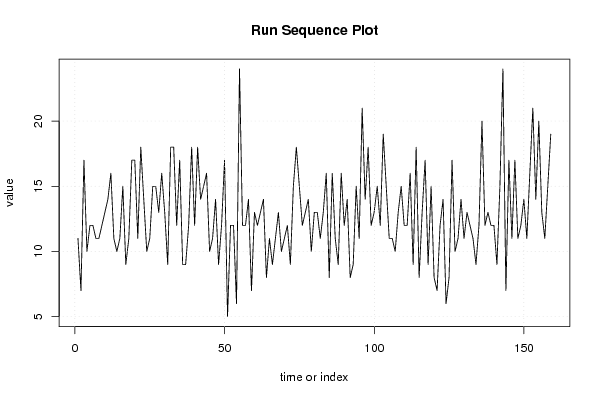PNG link Postscript link PDF link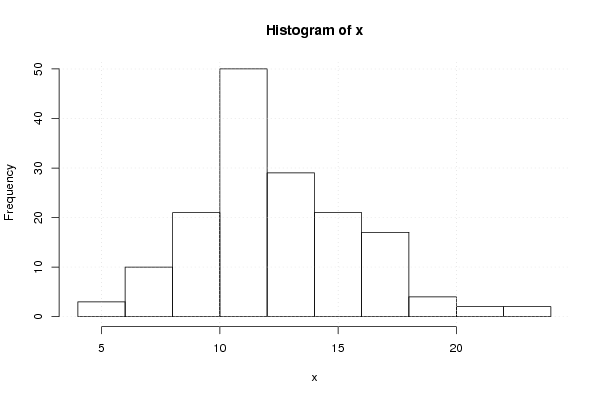PNG link Postscript link PDF link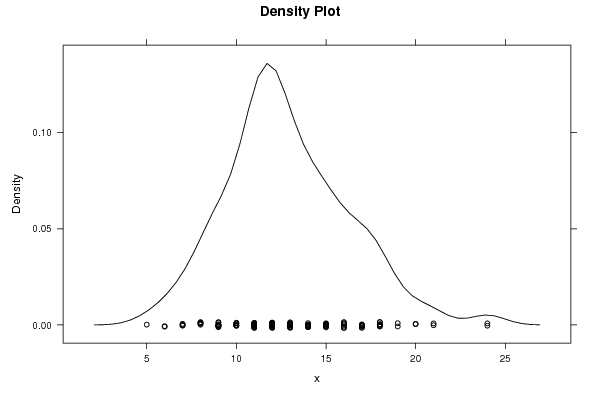PNG link Postscript link PDF link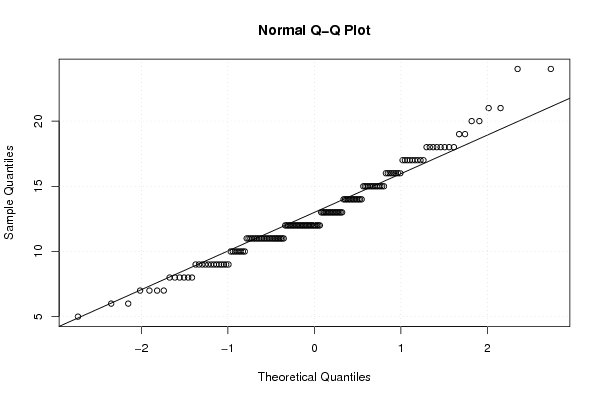PNG link Postscript link PDF link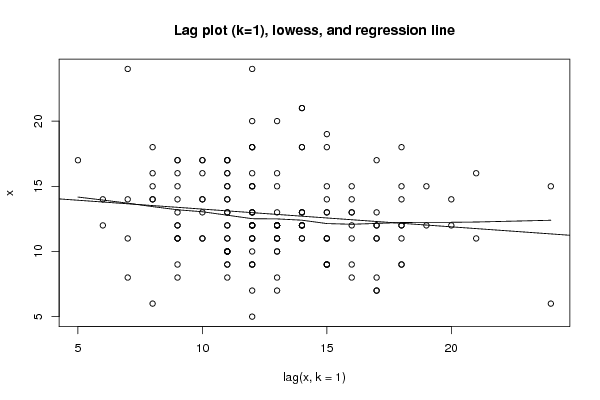PNG link Postscript link PDF link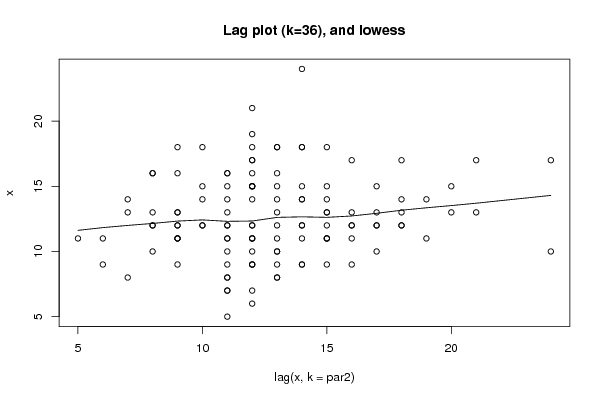PNG link Postscript link PDF link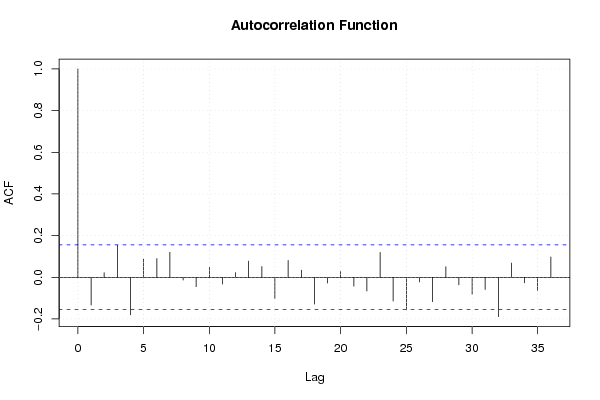PNG link Postscript link PDF link

Parameters (Session):
par1 = 0 ; par2 = 36 ;
Parameters (R input):
par1 = 0 ; par2 = 36 ;
R code (references can be found in the software module):
par1 <- as.numeric(par1)par2 <- as.numeric(par2)x <- as.ts(x)library(lattice)bitmap(file='pic1.png')plot(x,type='l',main='Run Sequence Plot',xlab='time or index',ylab='value')grid()dev.off()bitmap(file='pic2.png')hist(x)grid()dev.off()bitmap(file='pic3.png')if (par1 > 0){densityplot(~x,col='black',main=paste('Density Plot   bw = ',par1),bw=par1)} else {densityplot(~x,col='black',main='Density Plot')}dev.off()bitmap(file='pic4.png')qqnorm(x)qqline(x)grid()dev.off()if (par2 > 0){bitmap(file='lagplot1.png')dum <- cbind(lag(x,k=1),x)dumdum1 <- dum[2:length(x),]dum1z <- as.data.frame(dum1)zplot(z,main='Lag plot (k=1), lowess, and regression line')lines(lowess(z))abline(lm(z))dev.off()if (par2 > 1) {bitmap(file='lagplotpar2.png')dum <- cbind(lag(x,k=par2),x)dumdum1 <- dum[(par2+1):length(x),]dum1z <- as.data.frame(dum1)zmylagtitle <- 'Lag plot (k='mylagtitle <- paste(mylagtitle,par2,sep='')mylagtitle <- paste(mylagtitle,'), and lowess',sep='')plot(z,main=mylagtitle)lines(lowess(z))dev.off()}bitmap(file='pic5.png')acf(x,lag.max=par2,main='Autocorrelation Function')grid()dev.off()}summary(x)load(file='createtable')a<-table.start()a<-table.row.start(a)a<-table.element(a,'Descriptive Statistics',2,TRUE)a<-table.row.end(a)a<-table.row.start(a)a<-table.element(a,'# observations',header=TRUE)a<-table.element(a,length(x))a<-table.row.end(a)a<-table.row.start(a)a<-table.element(a,'minimum',header=TRUE)a<-table.element(a,min(x))a<-table.row.end(a)a<-table.row.start(a)a<-table.element(a,'Q1',header=TRUE)a<-table.element(a,quantile(x,0.25))a<-table.row.end(a)a<-table.row.start(a)a<-table.element(a,'median',header=TRUE)a<-table.element(a,median(x))a<-table.row.end(a)a<-table.row.start(a)a<-table.element(a,'mean',header=TRUE)a<-table.element(a,mean(x))a<-table.row.end(a)a<-table.row.start(a)a<-table.element(a,'Q3',header=TRUE)a<-table.element(a,quantile(x,0.75))a<-table.row.end(a)a<-table.row.start(a)a<-table.element(a,'maximum',header=TRUE)a<-table.element(a,max(x))a<-table.row.end(a)a<-table.end(a)table.save(a,file='mytable.tab')